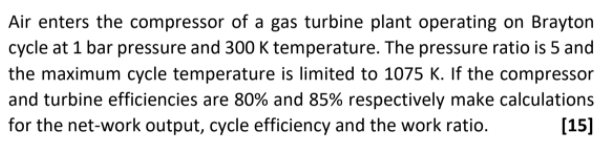# Air enters the compressor of a gas turbine plant operating on Brayton cycle at 1 bar pressure and 300 K temperature. The pressure ratio is 5 and the maximum cycle temperature is limited to 1075 K. If the compressor and turbine efficiencies are 80% and 85% respectively make calculations for the net-work output, cycle efficiency and the work ratio.  he-maximum-cycle-temperature-is-limited-to-1075-k-if

Question-AnswerCategory: QuestionsAir enters the compressor of a gas turbine plant operating on Brayton cycle at 1 bar pressure and 300 K temperature. The pressure ratio is 5 and the maximum cycle temperature is limited to 1075 K. If the compressor and turbine efficiencies are 80% and 85% respectively make calculations for the net-work output, cycle efficiency and the work ratio.  he-maximum-cycle-temperature-is-limited-to-1075-k-if

### Air enters the compressor of a gas turbine plant operating on Brayton cycle at 1 bar pressure and 300 K temperature. The pressure ratio is 5 and the maximum cycle temperature is limited to 1075 K. If the compressor and turbine efficiencies are 80% and 85% respectively make calculations for the net-work output, cycle efficiency and the work ratio.